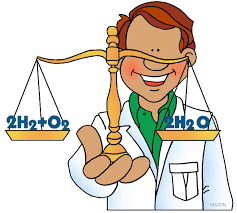# Acids and Bases: Balancing Equations

in prameshtyagi •  2 years ago

Acids react with metals, bases and carbonates to form salts. The equations are very important to know and there is a pattern that you can learn to follow.1.Acid + Metal → Salt + Hydrogen

For example:
2HCl(aq) + Zn(s) → ZnCl2(aq) + H2(g)

It's ionic net equation is:
2H+(aq) + Zn(s) → Zn2+(aq) + H2(g)

2.Acid + Base → Salt + Water

For example:
HCl(aq) + NaOH(aq) → NaCl(aq) + H2O(l)

It is a neutralization equation and the net ionic equation for any neutralization reaction is:
H+(aq) + OH–(aq) → H2O(l)

3.Acid + Carbonate → Salt + Water + Carbon dioxide

For example:
2HCl(aq) + CaCO3(s) → CaCl2(aq) + H2O(l) + CO2(g)

It's ionic net equation is:
CaCO3(s) + 2H+(aq) → Ca+(s) + H2O(l) + CO2(g)

In my exam I will probably get 3-4 reactions whose equations I will have to write, balance and then give ionic equations. I can easily gain marks in such questions as I just need to remember the products formed.

Sort Order:
·  2 years ago

see this post i memorized my school days, what a days

·  2 years ago

That chemical equations are oxidation and return. I lost or acquire protons, or loss
, The acquisition of electrons, the process is done together to obtain new outputs @
@renutyagi

·  2 years ago

Good little intro to sum simple chem.

Thanks :)

·  2 years ago

During studies earlier, I had many chemical reactions to remember. this post brought me back at the time, it was very interesting :) I wish you luck on the exam :)